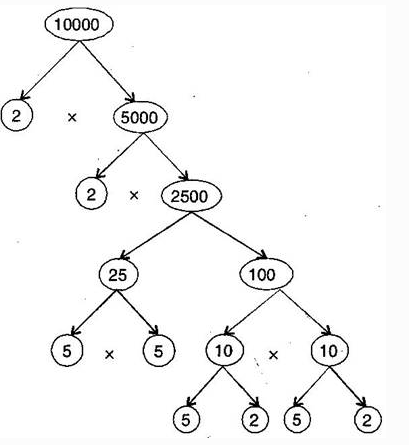Courses
Courses for Kids
Free study material
Free LIVE classes
MoreLIVE
Join Vedantu’s FREE Mastercalss

# Write all the smallest 5 digit numbers. Express it in terms of its prime factor by tree diagram.Verified
360.9k+ views
Hint: Prime factorization is a way of writing a given number as the product of its prime factors.
We know that smallest five digit number = 10000

Prime factor of 10000 = $$2 \times 2 \times 2 \times 2 \times 5 \times 5 \times 5 \times 5$$
Image of the factor tree:Note:
Prime factorization is finding which prime numbers multiply together to make the original number. The above tree diagram shows the process of breaking 10000 till we get all prime numbers at the end.
Last updated date: 19th Sep 2023
Total views: 360.9k
Views today: 7.60k